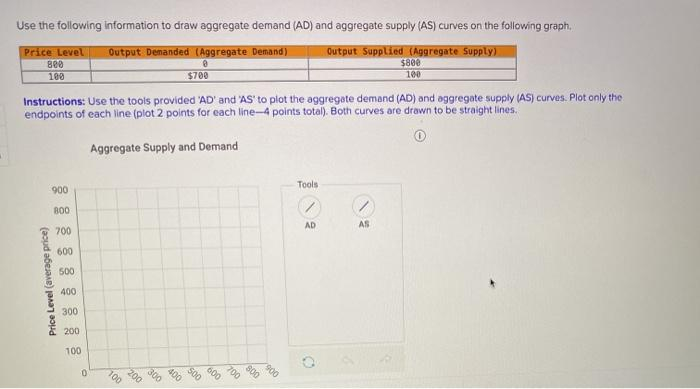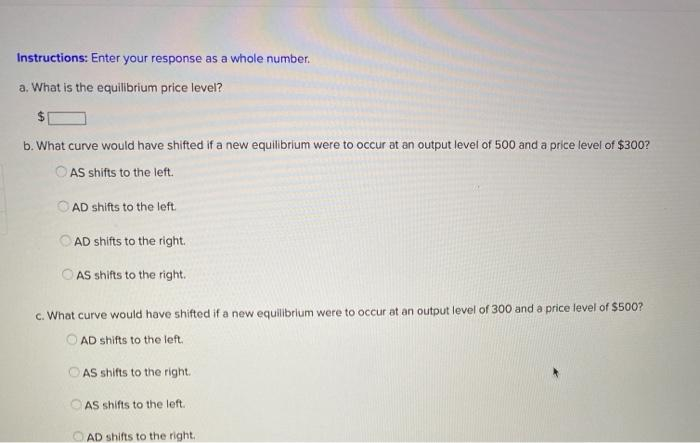### Create an Account

Already have account?

### Forgot Your Password ?

Home / Questions / Use the following information to draw aggregate demand (AD) and aggregate supply (AS) curv...

# Use the following information to draw aggregate demand (AD) and aggregate supply (AS) curves on the following graph, Price Level Output Demanded (Aggregate Demand) Output Supplied (Aggregate Supply

Use the following information to draw aggregate demand (AD) and aggregate supply (AS) curves on the following graph, Price Level Output Demanded (Aggregate Demand) Output Supplied (Aggregate Supply) 880 100 Instructions: Use the tools provided 'AD' and 'AS' to plot the aggregate demand (AD) and aggregate supply (AS) curves. Plot only the endpoints of each line (plot 2 points for each line-4 points total). Both curves are drawn to be straight lines. @ \$700 \$800 100 Aggregate Supply and Demand 900 Tools 800 AD AS 700 600 500 Price Level (average price) 400 300 200 100 0 100 300 200 006 900 7000 700 300
Instructions: Enter your response as a whole number. a. What is the equilibrium price level? b. What curve would have shifted if a new equilibrium were to occur at an output level of 500 and a price level of \$300? AS shifts to the left. AD shifts to the left AD shifts to the right AS shifts to the right c. What curve would have shifted if a new equilibrium were to occur at an output level of 300 and a price level of \$500? AD shifts to the left AS shifts to the right AS shifts to the left. AD shifts to the rightApr 12 2021 View more View Less

#### Answer (Solved)Subscribe To Get Solution